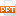# Bài giảng An Introduction to Computer Science Using Java - Chapter 9 Nested Loops and Two-Dimensional Arrays

Tài liệu Bài giảng An Introduction to Computer Science Using Java - Chapter 9 Nested Loops and Two-Dimensional Arrays: Chapter 9 Nested Loops and Two-Dimensional ArraysLecture Slides to AccompanyAn Introduction to Computer Science Using Java (2nd Edition)byS.N. Kamin, D. Mickunas, E. ReingoldChapter PreviewIn this chapter we will:show how nested loops are usefulintroduce two-dimensional arraysdescribe the use of two-dimensional arrays to represent grids of informationshow how computer graphics are generated using pixelsNested for LoopsNested loops frequently used to process two-dimensional arraysOften body of inner loop is where the main computation is doneExample:for (i = 0; i 0 yi , otherwiseDraw a pixel at (xi+1, yi+1)Compute pi = pi + 2y - 2x(yi+1 - yi)Two-Dimensional Arrays and lengthA.length is number of rows in two-dimensional array AA[i].length is number of columns in row i from two-dimensional array AIn Java rows can be of different lengthsExample:int[][] A = new int[];for (int i = 0; i < 5; i++) { A[i] = new int[i + 1];}...17 trang | Chia sẻ: honghanh66 | Ngày: 19/03/2018 | Lượt xem: 315 | Lượt tải: 0
Bạn đang xem nội dung tài liệu Bài giảng An Introduction to Computer Science Using Java - Chapter 9 Nested Loops and Two-Dimensional Arrays, để tải tài liệu về máy bạn click vào nút DOWNLOAD ở trên
Chapter 9 Nested Loops and Two-Dimensional ArraysLecture Slides to AccompanyAn Introduction to Computer Science Using Java (2nd Edition)byS.N. Kamin, D. Mickunas, E. ReingoldChapter PreviewIn this chapter we will:show how nested loops are usefulintroduce two-dimensional arraysdescribe the use of two-dimensional arrays to represent grids of informationshow how computer graphics are generated using pixelsNested for LoopsNested loops frequently used to process two-dimensional arraysOften body of inner loop is where the main computation is doneExample:for (i = 0; i 0 yi , otherwiseDraw a pixel at (xi+1, yi+1)Compute pi = pi + 2y - 2x(yi+1 - yi)Two-Dimensional Arrays and lengthA.length is number of rows in two-dimensional array AA[i].length is number of columns in row i from two-dimensional array AIn Java rows can be of different lengthsExample:int[][] A = new int[];for (int i = 0; i < 5; i++) { A[i] = new int[i + 1];}

Các file đính kèm theo tài liệu này:

•chapter9_9572.ppt
Tài liệu liên quan